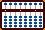###Conversion from Decimal to Binary - by Marcos Labriola

Hello People at SorobanAbacus

I would like to share with you a method to convert Decimal to Binary I devised long ago.

#### Conversion from Decimal to Binary

The procedure consists of the repeated application of three steps for each digit of the decimal number we wish to convert, from left (most significant) to right (least significant). It is based on the positional representation of decimal numbers and is entirely performed using binary arithmetic:

Clear the abacus
For each decimal digit, from left to right
step 1: Multiply the existing binary number by ten
step 2: Add the binary representation of the decimal digit
step 3: Normalize

Let us detail each step.

#### Step 1

The first step need not be performed for the first decimal digit, since the abacus is clean.

It is done by the substitution of every binary digit 1 by two digits 1, the first immediately to the left and the second in the third position to the left. For instance,

 0000000000100 is replaced by 0000000101000 0000000000111 is replaced by 0000000112110 (4) (40) (7) (70 not normalized)

The substitutions are best performed from left to right, to avoid confusion. For instance,

 0000000000111 0000000101011 0000000111101 0000000112110 (7) (70 not normalized)

Notice that we are temporarily allowed to have digits bigger than 1 in our binary representations because they will be normalized later.

#### Step 2

Next, we add the current decimal digit, not being concerned with carries. For instance, suppose the next decimal digit is five, which is 101 in binary.

 0000000112110 becomes 0000000112211 (70 not normalized) (75 not normalized)

#### Step 3

We normalize to get the final result or prepare for the next decimal digit. Normalization is performed from right to left by substitution of every two beads from current position with one bead on next position. Following the example above, we have:

 0000000112211 0000000113011 0000000121011 0000000201011 0000001001011 (75 not normalized) (75)

======

#### Finally, suppose we are converting 752 to binary, continuing from previous example:

 0000001001011 0001010001011 0001011010011 0001011020101 0001011021110 0001011021120 0001011021200 0001011022000 0001011030000 0001011110000 (75) (750 not normalized) (752 not normalized)   (752 normalized)

=======
Hope you enjoy. Once you master it, you can convert quickly.

Marcos